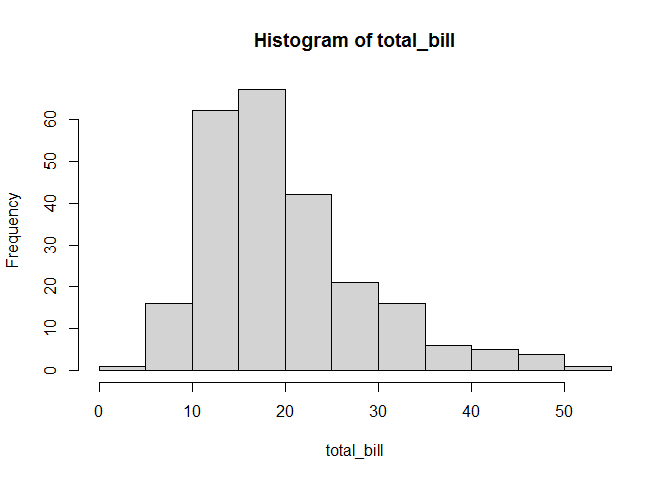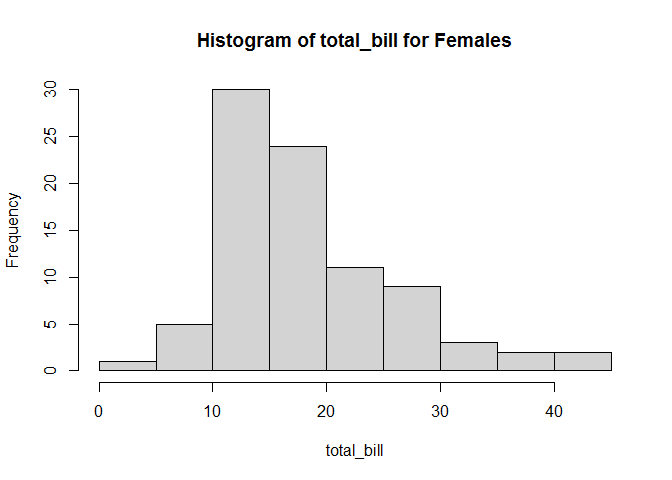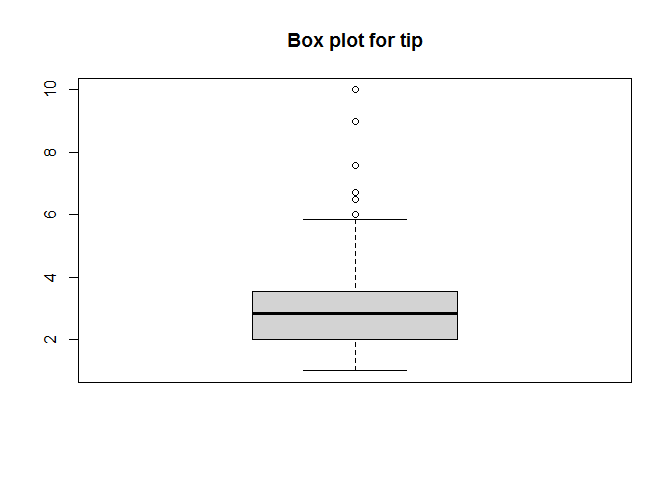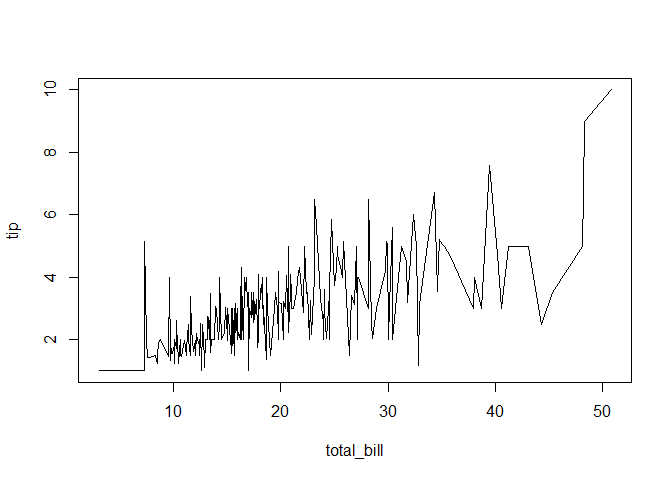# Getting Started with R Programming Language: Exercises and Solutions

These exercises aim to review the basics of R. The following R practice questions include (1) reading tabular data, (2) handling missing values, (3) filtering data with subsets, (4) simple graphing, and (5) correlations.

In order to solve the tasks you need:

D0 <- read.csv("Tips.csv")

## 2. Data Inspection

1. Show the brief description and summary statistics for the imported data using str() and summary().
str(D0)
## 'data.frame':    244 obs. of  7 variables:
##  $total_bill: num 17 10.3 21 23.7 24.6 ... ##$ tip       : num  1.01 1.66 3.5 3.31 3.61 4.71 2 3.12 1.96 3.23 ...
##  $sex : chr "Female" "Male" "Male" "Male" ... ##$ smoker    : chr  "No" "No" "No" "No" ...
##  $day : chr "Sun" "Sun" "Sun" "Sun" ... ##$ time      : chr  "Dinner" "Dinner" "Dinner" "Dinner" ...
##  $size : int 2 3 3 2 4 4 2 NA 2 2 ... summary(D0) ## total_bill tip sex smoker ## Min. : 3.07 Min. : 1.000 Length:244 Length:244 ## 1st Qu.:13.32 1st Qu.: 2.000 Class :character Class :character ## Median :17.81 Median : 2.900 Mode :character Mode :character ## Mean :19.81 Mean : 2.998 ## 3rd Qu.:24.18 3rd Qu.: 3.562 ## Max. :50.81 Max. :10.000 ## NA's :1 ## day time size ## Length:244 Length:244 Min. :1.000 ## Class :character Class :character 1st Qu.:2.000 ## Mode :character Mode :character Median :2.000 ## Mean :2.568 ## 3rd Qu.:3.000 ## Max. :6.000 ## NA's :1 1. Show the number of rows and columns using nrow() and ncol(). # number of rows nrow(D0) ##  244 # number of columns ncol(D0) ##  7 1. Inspect whether there exist missing values. If do, remove the row(s) of missing values using is.na() and na.omit(). Name the new data set D1. sum(is.na(D0)) ##  3 There are 3 missing values in the data set. D1 <- na.omit(D0) 1. Repeat part (a) for D1 and compare the results with (a). str(D1) ## 'data.frame': 241 obs. of 7 variables: ##$ total_bill: num  17 10.3 21 23.7 24.6 ...
##  $tip : num 1.01 1.66 3.5 3.31 3.61 4.71 2 1.96 1.71 5 ... ##$ sex       : chr  "Female" "Male" "Male" "Male" ...
##  $smoker : chr "No" "No" "No" "No" ... ##$ day       : chr  "Sun" "Sun" "Sun" "Sun" ...
##  $time : chr "Dinner" "Dinner" "Dinner" "Dinner" ... ##$ size      : int  2 3 3 2 4 4 2 2 2 4 ...
##  - attr(*, "na.action")= 'omit' Named int [1:3] 8 10 240
##   ..- attr(*, "names")= chr [1:3] "8" "10" "240"
summary(D1)
##    total_bill         tip             sex               smoker
##  Min.   : 3.07   Min.   : 1.000   Length:241         Length:241
##  1st Qu.:13.28   1st Qu.: 2.000   Class :character   Class :character
##  Median :17.78   Median : 2.830   Mode  :character   Mode  :character
##  Mean   :19.74   Mean   : 2.985
##  3rd Qu.:24.06   3rd Qu.: 3.550
##  Max.   :50.81   Max.   :10.000
##      day                time                size
##  Length:241         Length:241         Min.   :1.000
##  Class :character   Class :character   1st Qu.:2.000
##  Mode  :character   Mode  :character   Median :2.000
##                                        Mean   :2.568
##                                        3rd Qu.:3.000
##                                        Max.   :6.000

There are less observations in the D1 data frame (241, compared to 244 in D0 data frame) and there are no NA’s in D1 data frame.

## 3. Data Subset

1. Subset data D1 with respect to the condition that column “time” is equal to “Dinner”. Name the derived data frame as D2.
D2 <- subset(D1, time == "Dinner")
1. Remove data set D2 using rm().
rm(D2)

## 4. Graphing

1. Plot histogram for the total_bill from D1.
hist(D1$total_bill, main = "Histogram of total_bill", xlab = "total_bill")1. Plot histogram for the total_bill for Females from D1. hist(D1$total_bill[D1$sex == "Female"], main = "Histogram of total_bill for Females", xlab = "total_bill")1. Plot box plot for tip from D1. boxplot(D1$tip, main = "Box plot for tip")## 5. Correlations

1. Calculate mean and variance for total_bill from D1.
# mean
mean(D1$total_bill) ##  19.73892 # variance var(D1$total_bill)
##  79.57122
1. Calculate the correlation between total_bill and tip from D1.
cor(D1$total_bill, D1$tip)
##  0.6758822
1. Draw a line plot where total_bill as x-axis, tip as y-axis using plot().
# sort observations by total_bill
D2 <- D1[order(D1$total_bill), ] # line plot plot(D2$total_bill, D2\$tip, type = "l", xlab = "total_bill", ylab = "tip")1. Compare the correlation value and line plot, report your observations.

Correlation value of 0.676 suggests that there is a strong positive relationship between total_bill and tip (as total_bill increases, tip also tends to increase). The same relationship is also visible in the line plot.